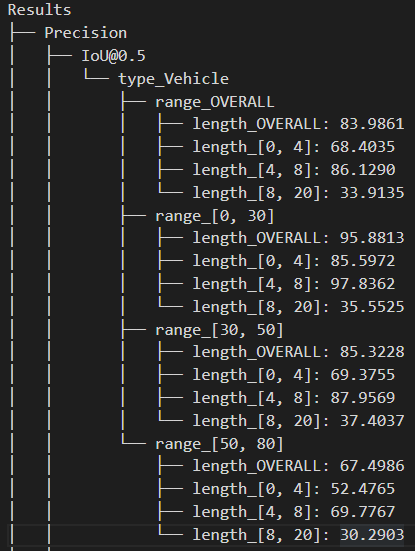# A simple and extensible object detection evaluator in COCO style

## Features

• Extensible user interfaces to deal with different data formats.
• Support customized evaluation breakdowns, e.g., object size in COCO, difficulty in KITTI, velocity and range in Waymo.
• Interface for general matching scores, e.g. 2D IoU, 3D rotated IoU, center distance.
• Support widely-used metrics like AP, AR and customized metrics like average regression errors, average IoUs, etc.
• Purely based on Python, easy to develop your customized metrics.

## Installation

• `pip install treelib`
• Clone this repo and run `pip install .` in the cloned directory, or `pip install -e .` if you want to modify the source code.

## Prepare predictions and groundtruth

You need to define a function to read the predictions and groundtruth.

``````def read_prediction(path):
...
return results
``````

where the `results` is a dictionary using sample_id (image_id) as key and each item is a dictionary contains at least `box`, `score` and `type`:

``````# ndarray in shape of [N, C], where N is the number of bboxes in this sample and C is the box dimension
boxes = ...

# ndarray in shape [N,]
scores = ...

# ndarray in shape of [N,]
types = ...

results[sample_id] = dict(box=boxes, score=scores, type=types)
``````

And you need to define another function to read groundtruth as the same way. The items of returned dict contain at least `box` and `type`.

## Customize matching score calculator

If you are going to evaluate a 2D detector, you may want to define a 2D IoU function, which will be automatically used in the matching process.

``````def iou_2d(box1, box2):
# box1 in shape of [N1, 4], which is the box item defined above.
# box2 in shape of [N2, 4], which is the box item defined above.

iou_matrix = ... # [N1, N2]
return iou_matrix
``````

You are free to define any other matching score calculators (e.g., 3D IoU), as long as keeping the same function prototype. For example:

``````def customized_iou_calculator(box1, box2):
# box1 in shape of [N1, box_dim]
# box2 in shape of [N2, box_dim]

iou_matrix = ... # [N1, N2]
return iou_matrix
``````

## Customize breakdowns

To correctly use customized breakdowns, here we define two kinds of breakdowns: `separable breakdowns` and `inseparable breakdowns`.

`Separable breakdowns` indicate those can be used to partition prediction set and groundtruth set before the matching process. For example, `object category` is a typical separable breakdown. We usually first partition the predictions and groundtruth and only pass the predictions and groundtruth in the same category to the evaluator.

`Inseparable breakdowns` indicate those can NOT be simply used to partition prediction set and groundtruth set before the matching process. A typical inseparable breakdown is `object size` in COCO. We deal with those inseparable breakdowns in the matching process.

### 1. Define breakdown functions

For example, if you want to evaluate conditioned on vehicle length in Waymo:

``````def waymo_length_breakdown(object_item):
# the object item is defined in Sec. prepare-predictions-and-groundtruth
return object_item['box'][:, 4] # 4th number indicates vehicle length
``````

### 2. Define breakdown values

If you want to add category and size as breakdowns in COCO:

``````def get_object_type(object_item):
return object_item['type'] # 4th number indicates vehicle length

def get_object_size(object_item):
box = object_item['box']
return (box[:, 2] * box[:, 3]) ** 0.5  # height x width

separable_breakdown_dict = {'type': [None, 'person', 'bus']} # None means evaluate over all categories.
inseparable_breakdown_dict = {'size': [None, (0, 32), (32, 96), (96, 1e10)]} # None means evaluate over all sizes.

breakdown_func_dict = {'type': get_object_type, 'size': get_object_size}
``````

## Launch evaluation

### 1. Put the pre-defined stuff into a params object

For example in Waymo:

``````from od_evaluation.params import BaseParam
class WaymoBaseParam(BaseParam):

def __init__(self, pd_path, gt_path, interval=10, update_sep=None, update_insep=None):
super().__init__(pd_path, gt_path, interval, update_sep, update_insep)
self.iouThrs = [0.7, 0.5]

self.separable_breakdowns = {
'type':('Vehicle', 'Pedestrian', 'Cyclist'),
'range':([0, 30], [30, 50], [50, 80], None), # None means the union of all ranges
}
self.inseparable_breakdowns = {'length':[(0, 4), (4, 8), (8, 20)]}
self.breakdown_func_dict = {'range': get_waymo_object_range, 'length': get_waymo_object_length}

self.iou_calculate_func = get_waymo_iou_matrix

``````

Note that you must implement `add_breakdowns`, `add_iou_function` and `add_input_function` after inherit `BaseParam`.

### 2. Begin evaluation

``````from od_evaluation.eval import Evaluator
pd_path = xxx
gt_path = xxx
params = WaymoBaseParam(pd_path, gt_path)
e = Evaluator(params)
e.run()
``````

## Output

We use treelib to format the evaluation results, where multiple breakdowns are nested:View Github